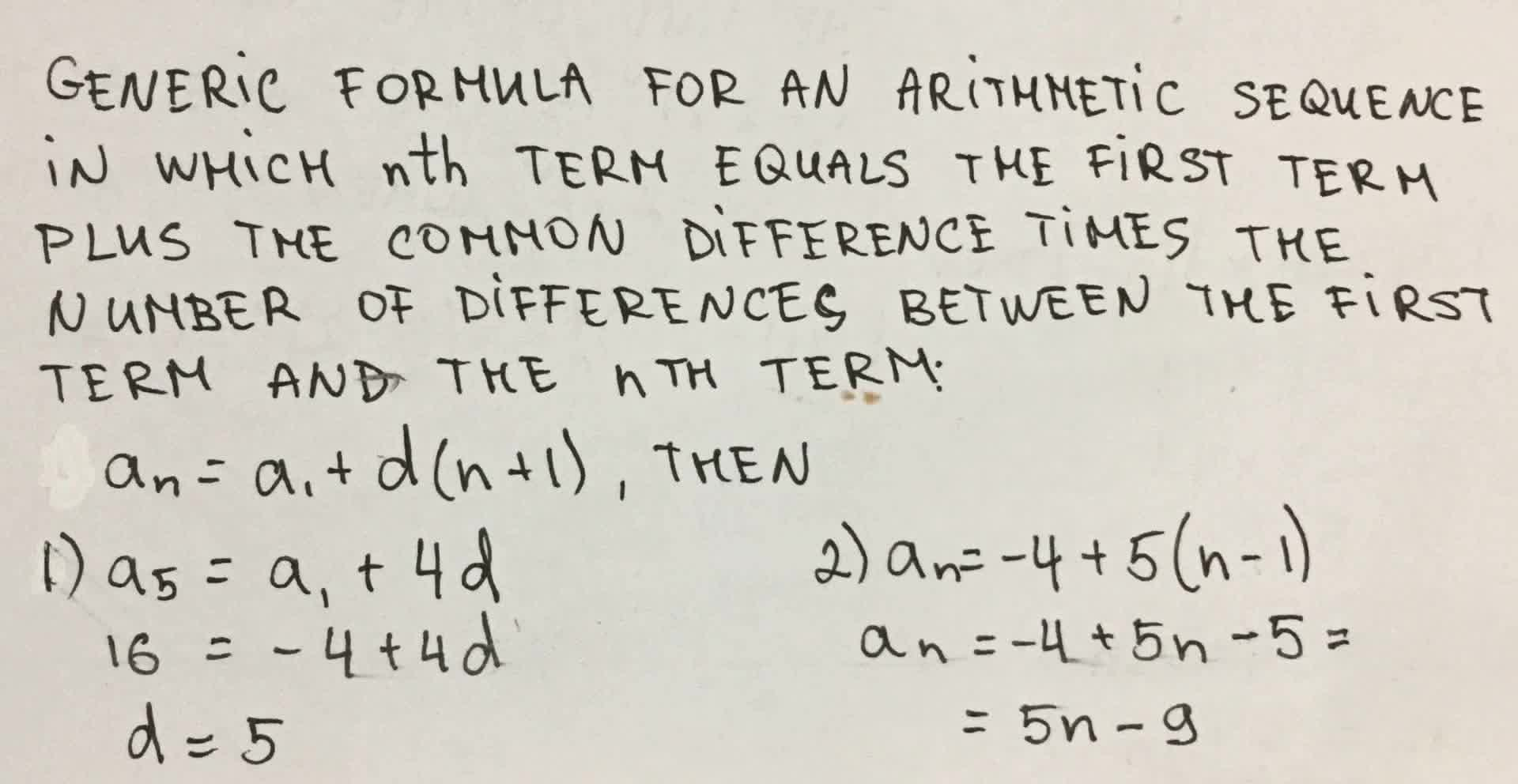# find a formula for an for the arithmetic sequence. a_1 = -4, a_5 = 16snowlovelydayM 2021-09-15 Answered
find a formula for an for the arithmetic sequence. $$\displaystyle{a}_{{1}}=-{4},{a}_{{5}}={16}$$

• Questions are typically answered in as fast as 30 minutes

### Plainmath recommends

• Get a detailed answer even on the hardest topics.
• Ask an expert for a step-by-step guidance to learn to do it yourself.Nathalie Redfern

Generic formula for an arithmetic sequence in which nth term equals the first term plus the common difference times the number of differences between the first term and the nth term:
$$\displaystyle{a}_{{n}}={a}_{{1}}+{d}{\left({n}-{1}\right)}$$, then
1) $$\displaystyle{a}_{{5}}={a}_{{1}}+{4}_{{d}}$$
$$\displaystyle{16}=-{4}+{4}_{{d}}$$
$$\displaystyle{d}={5}$$
2) $$\displaystyle{a}_{{n}}=-{4}+{5}{\left({n}–{1}\right)}$$
$$\displaystyle{a}_{{n}}=-{4}+{5}_{{n}}–{5}={5}_{{n}}-{9}$$

###### Have a similar question?content_user
Answer is given on photo